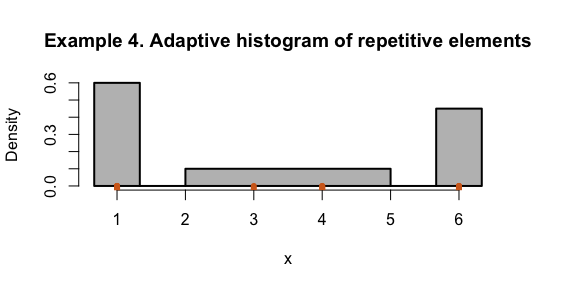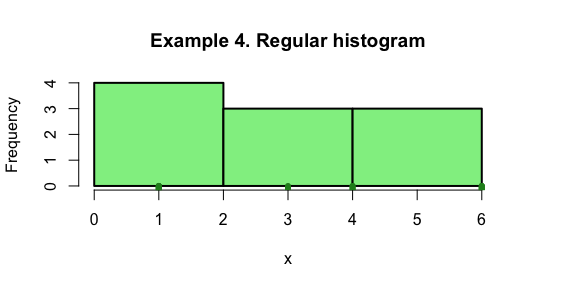# Tutorial: Adaptive versus regular histograms

#### Updated 2020-11-07

Adaptive histograms can reveal patterns in data more effectively than regular (equal-bin-width) histograms. The “discontinuous” style adaptive histogram is recommended because it adapts to the data without attempting to assign a non-zero density to every bin. When bins are not equal with, the vertical axis of the histogram is density instead of frequencies.

## Example 1: Data from a Gaussian mixture model using one bin per cluster

Plot an adaptive histogram from data generated by a Gaussian mixture model with three components:

require("Ckmeans.1d.dp")
x <- c(rnorm(40, mean=-2, sd=0.3),
rnorm(45, mean=1, sd=0.1),
rnorm(70, mean=3, sd=0.2))
ahist(x, col="lightblue", sub=paste("n =", length(x)),
col.stick="darkblue", lwd=2, xlim=c(-4,4),
main="Example 1. Gaussian mixture model with 3 components\n(one bin per component)\nAdaptive histogram")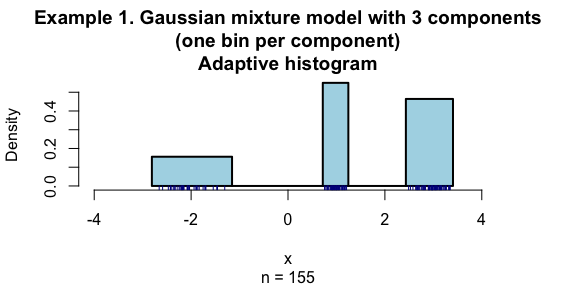When breaks is specified, ahist will call hist (regular histogram function in R).

ahist(x, breaks=3, col="lightgreen", sub=paste("n =", length(x)),
col.stick="forestgreen", lwd=2,
main="Example 1. Regular histogram")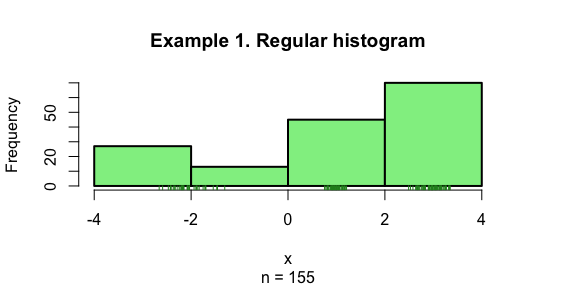## Example 2: Data from a Gaussian mixture model using three bins per cluster

Plot an adaptive histogram from data generated by a Gaussian mixture model with three components using a given number of bins

ahist(x, k=9, col="lavender", col.stick="navy",
sub=paste("n =", length(x)), lwd=2,
main="Example 2. Gaussian mixture model with 3 components\n(on average 3 bins per component)\nAdaptive histogram")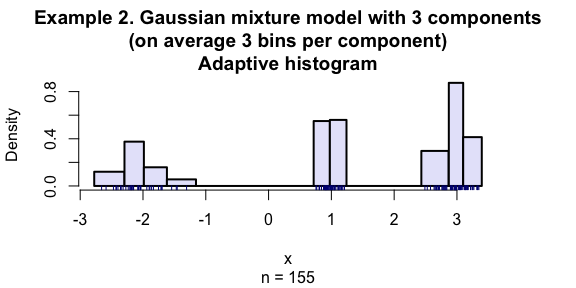When breaks is specified, ahist will call hist (regular histogram function in R).

ahist(x, breaks=9, col="lightgreen", col.stick="forestgreen",
sub=paste("n =", length(x)), lwd=2,
main="Example 2. Regular histogram")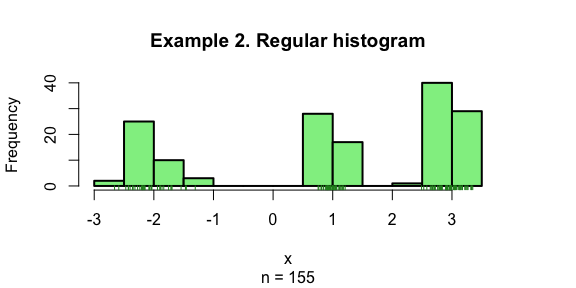## Example 3: Adaptive histogram of protein DNase

The DNase data frame has 176 rows and 3 columns of data obtained during development of an ELISA assay for the recombinant protein DNase in rat serum:

data(DNase)
res <- Ckmeans.1d.dp(DNase$density) kopt <- length(res$size)
ahist(res, data=DNase$density, col=rainbow(kopt), col.stick=rainbow(kopt)[res$cluster],
sub=paste("n =", length(x)), border="transparent",
xlab="Optical density of protein DNase",
main="Example 3. Elisa assay of DNase in rat serum\nAdaptive histogram")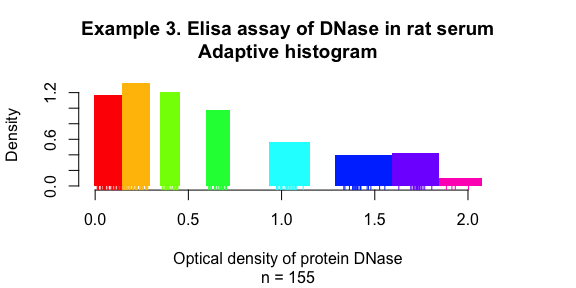Using the same data with Example 3, this example demonstrates the inadequacy of equal-bin-width histograms. The third bin gives a false sense of sample distribution.

We can specifiy breaks=“Sturges” in ahist() function to use equal-bin-width histograms. The difference is that sticks are added to the histogram by ahist(), but not by the R provided hist() function.

ahist(DNase\$density, breaks="Sturges", col="palegreen",
main="Example 3. Elisa assay of DNase in rat serum\nRegular histogram (equal bin width)",
xlab="Optical density of protein DNase")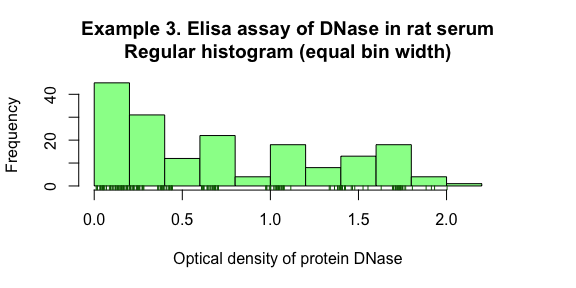## Example 4. Repetitive data

Cluster data with repetitive elements:

x <- c(1,1,1,1, 3,4,4, 6,6,6)
ahist(x, k=c(2,4), col="gray",
lwd=2, lwd.stick=6, col.stick="chocolate",
main="Example 4. Adaptive histogram of repetitive elements")
ahist(x, breaks=3, col="lightgreen",
lwd=2, lwd.stick=6, col.stick="forestgreen",
main="Example 4. Regular histogram")
## Warning in cluster.1d.dp(x, k, y, method, estimate.k, "L2", deparse(substitute(x)), : Max number of clusters is greater than the unique number of
## elements in the input vector, and k.max is set to the number of
## unique number of input values.